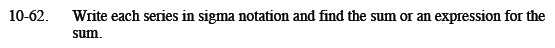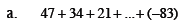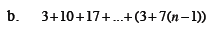Home > CCA2 > Chapter 10 > Lesson 10.1.4 > Problem10-62

10-62.
1. Write each series in sigma notation and find the sum or an expression for the sum. Homework Help ✎

1. 47 + 34 + 21 + … + (−83)

2. 3 + 10 + 17 + … + (3 + 7(n − 1))What is the expression for the sequence?

How many terms are there in the series?

$\sum_{k = 1}^{11}(60-13k)=-198$